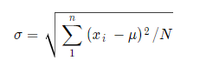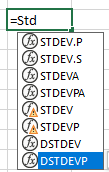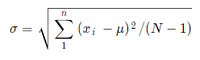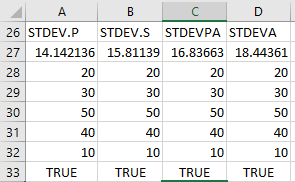# How To Calculate Standard Deviation in Excel?

• Last Updated : 09 May, 2021

In this article, we will learn about the calculation of the standard deviation(std) in Excel.

First, let’s learn about Standard Deviation. In real-life scenarios, we have populations like marks of students in a particular subject, salaries of multiple employees in a company. Let us consider, in a company named ABC, we have 5 employees.

Now, it will tell us that how these salaries are dispersed across this company.

The formula for std is –

\sigma =  \sqrt{ \sum_1^n (x_{i} -  \mu  )^{2}  /N}

It will be shown asWhere x represents the value, and μ is the mean of the population.

• Mean = 30
• N = 5
• Std = 14.14

Now, Let’s learn how to use it in Excel.

In Excel we have formulas for everything and as you might have guessed for std also. We can have this list of formulas using ‘=’ symbol shown belowLet’s talk about them briefly,

1. STDEV.P – It calculates the std assuming that the entire population is its argument. It returns an approximate value and is used with a large population. Uses the same formula shown above.

2. STDEV.S – It calculates the std assuming that the sample of the population is its argument. It returns an approximate value and is used with a large population. Uses the same formula shown below

\sigma =  \sqrt{ \sum_1^n (x_{i} -  \mu  )^{2}  / (N -1)}3. STDEVA – It includes text and logical symbols whereas the above 2 functions don’t include text and logical symbols. It is used for a sample of the population. Uses “n-1” formula.

4. STDEPA – It includes text and logical symbols. It is used for the whole population. Uses “n” formula.

Let’s see the difference here,Here, the discrepancy occurs because STDEVPA and STDEVA read TRUE as 1 and STDEV.P and STDEV.S ignore it.

The formula goes like this:

1. =STDEV.P(A28:A33)        [Formula used in A27 cell]
2. =STDEV.S(B28:B33)        [Formula used in B27 cell]
3. =STDEVPA(A28:A33)        [Formula used in C27 cell]
4. =STDEVA(A28:A33)         [Formula used in D27 cell]

Note – STDEV and STDEVP are for Excel 2007 or earlier. DSTDEV and DSTDEVP are used for fetching data from Database.

My Personal Notes arrow_drop_up